## 概念

### 算法的性质：有穷性、确定性、可行性

• 有穷性：算法必须在有限个计算步骤后终止。
• 确定性：算法必须是没有歧义的。
• 可行性：可以机械地一步一步执行基本操作步骤。

### 算法的分析

• 统一机器性能
• 分析最坏情况

#### 算法分析的工具——时间复杂度

• 渐近紧确界：用记号 $\theta$ 表示。对于给定的函数 $g(n)$$\theta(g(n))$ 表示以下函数的集合：$\theta(g(n))=\{ T(n):\exists c_1,c_2,n_0>0,使得\forall n\geq n_0,c_1g(n)\leq T(n)\leq c_2g(n)\}$$T(n)$ 的上界 $c_1g(n)$ 即是 $O$ ，下界即是 $\Omega$

• 渐近上界：用记号 $O$ 表示。对于给定的函数 $g(n)$$O(g(n))$ 表示以下函数的集合：$O(g(n))=\{T(n):\exists c,n_0>0,使得\forall n\geq n_0,0\leq T(n)\leq cg(n)\}$

• 渐近下界：用记号 $\Omega$ 表示。对于给定的函数 $g(n)$$\Omega(g(n))$ 表示以下函数的集合：$\Omega(g(n))=\{T(n):\exists c,n_0>0,使得\forall n\geq n_0, 0\leq cg(n)\leq T(n)\}$

$T(n)=\theta(g(n))$ 等价于：$T(n)=\Omega(g(n))$$T(n)=\Omega(g(n))$

## 分而治之法

### 归并排序

#### 算法流程：分解数组->递归求解->合并排序

• 分解数组：将数组$A[1,n]$排序问题分解为 $A[1,\lfloor \frac{n}{2} \rfloor]$$A[\lfloor \frac{n}{2} \rfloor+1,n]$ 排序问题。
• 求解：递归解决子问题得到两个有序的子数组
• 排序：将两个有序子数组合并为一个有序数组

#### 伪代码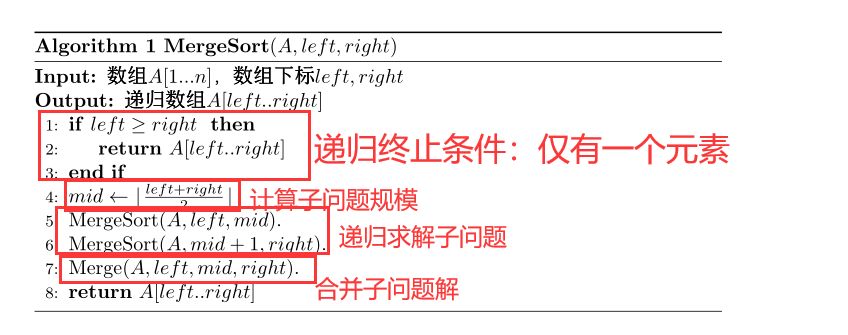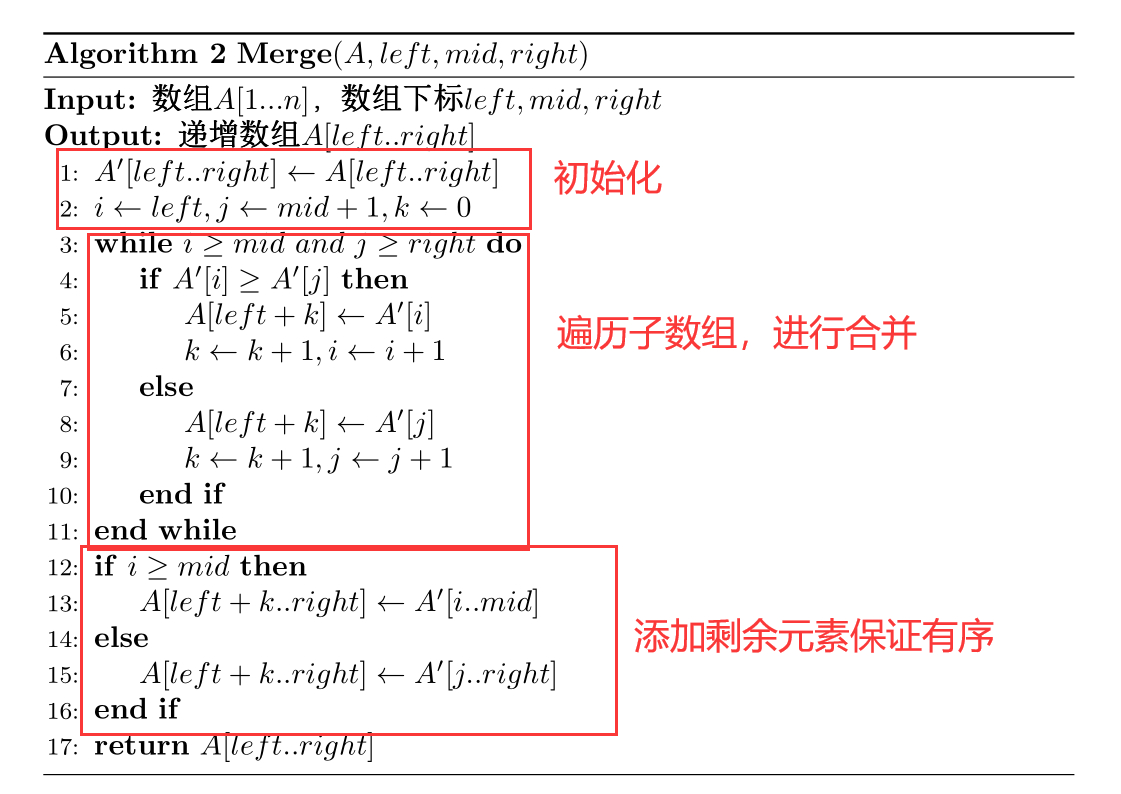#### 复杂度分析

$T(n)$：完成 $MergeSort(A,1,n)$ 的运行时间。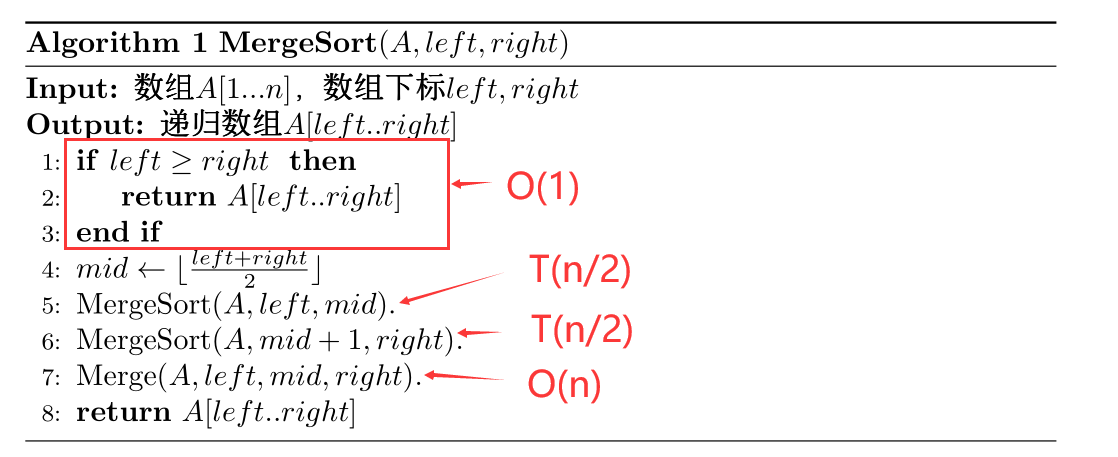$T(n)=\left\{ \begin{array}{lr} 2T(n/2)+O(n), & if\ n>1 \\ O(1), & if\ n=1 \end{array} \right.$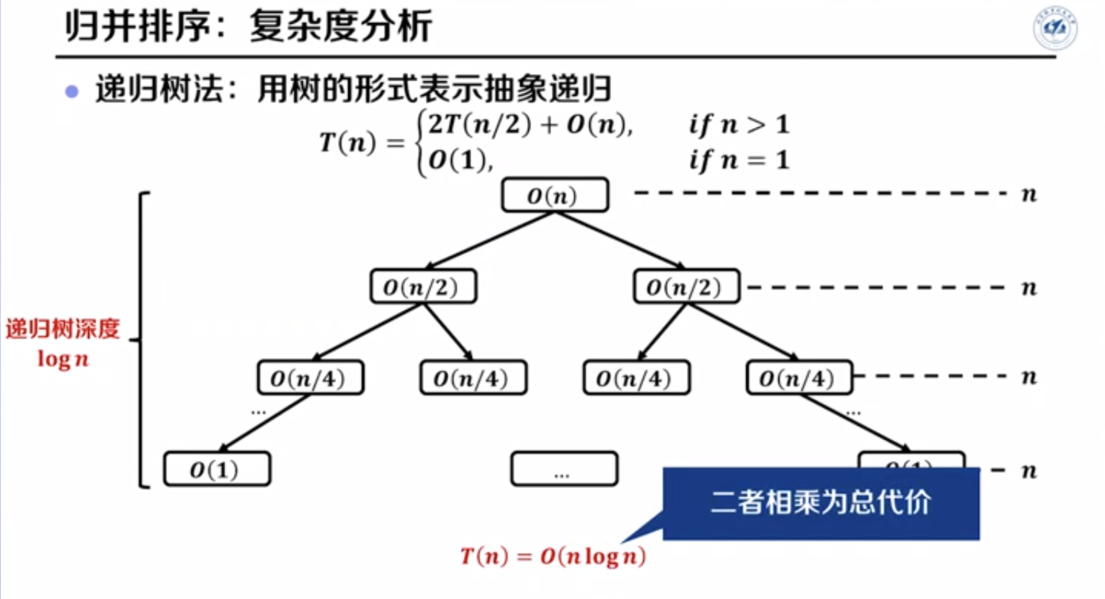### 最大子数组问题

#### 问题定义

$S(l,r)=\sum_{i=l}^rX[i]$

#### 蛮力枚举法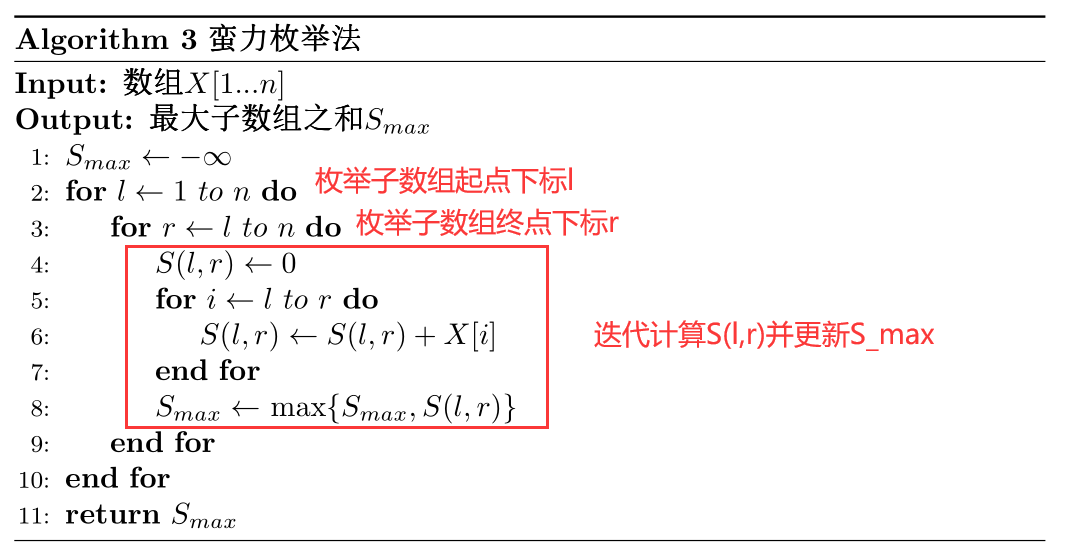#### 优化枚举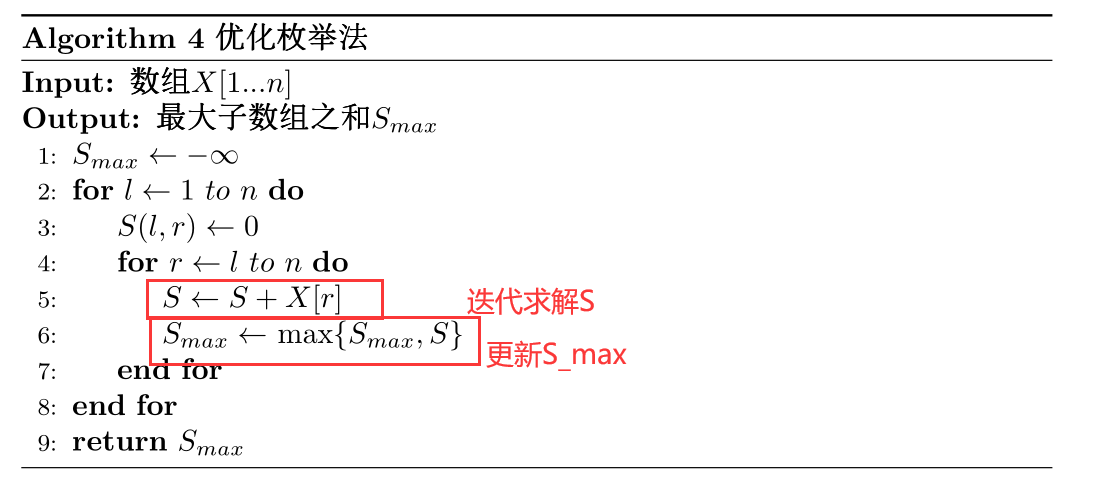#### 分而治之

• 将数组 $X[1..n]$ 分为 $X[1..n/2]$$X[n/2+1..n]$
• 递归求解子问题
• $S_1$：数组 $X[1..n/2]$ 的最大子数组
• $S_2$：数组 $X[n/2+1..n]$ 的最大子数组
• 合并子问题，得到$S_{max}$
• $S_3$：跨中点的最大子数组
• 数组 $X$ 的最大子数组之和 $S_{max}=max\{S_1,S_2,S_3\}$

• $mid=n/2$
• $S_3$可以分为左右两部分
• $Left$：以 $X[mid]$ 为结尾的最大子数组之和
• $Right$：以 $X[mid+1]$ 为开头的最大子数组之和
• $S_3=Left+Right$
• 求解$Left$
• $mid=n/2$
• $X[mid]$ 向前遍历求和，并记录最大值
• $X[mid]$ 为结尾的最大子数组之和 $S_{left}=4$
• 求解$Right$
• $mid=n/2$
• $X[mid+1]$ 向后遍历求和，并记录最大值
• 时间复杂度：$T(n)=T(Left)+T(Right)=O(mid)+O(n-mid)=O(n)$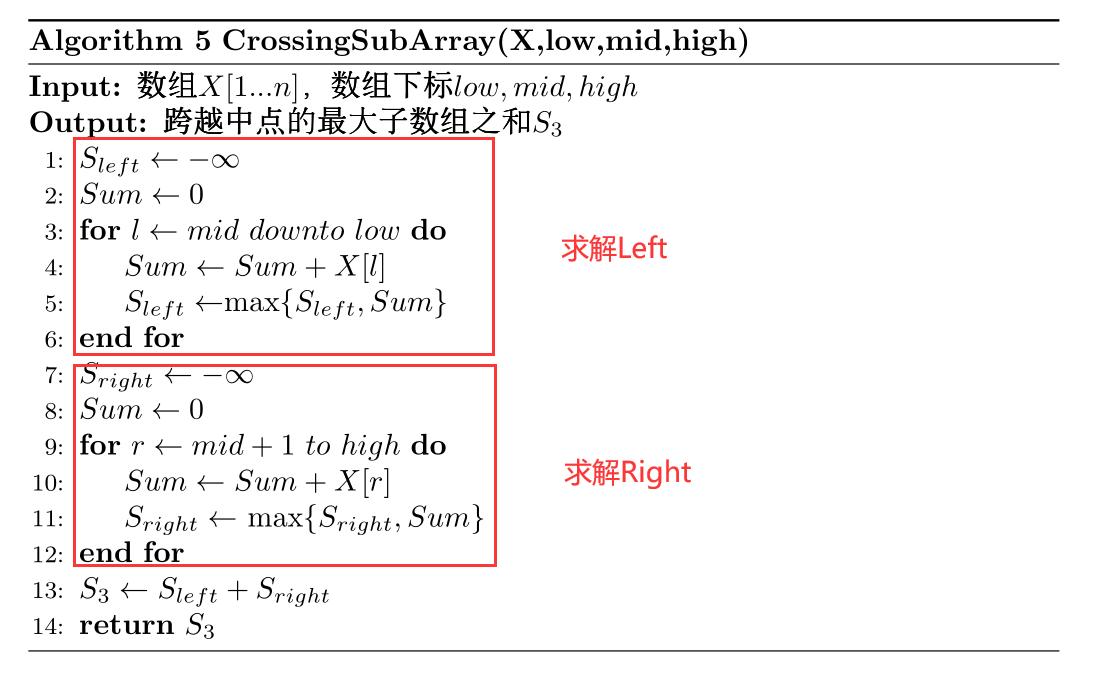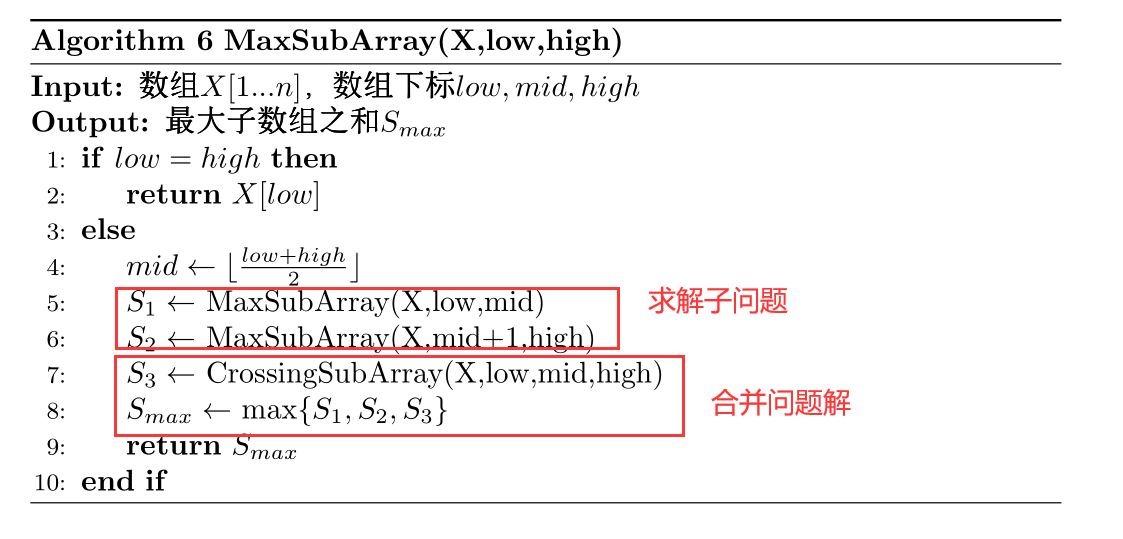• 输入规模：$n$

• $T(n)=\left\{ \begin{array}{lr} 1, & n=1 \\ 2\cdot T(n/2)+O(n), & n >1 \end{array} \right.$

• 时间复杂度 $O(n\log n)$